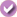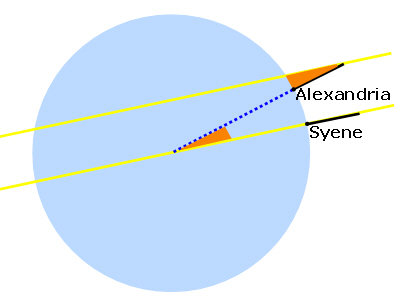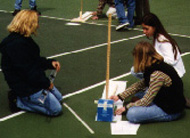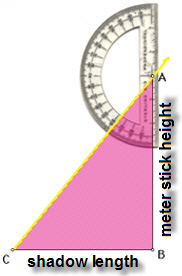# Calculating the Circumference of the Earth

 Areas of Science Astronomy Difficulty Time Required Short (2-5 days) Prerequisites You will need to understand some basic principles of geometry for this project. You will need a friend or relative in a distant city to make a shadow measurement for you on the same day you make yours. Both of you will need clear weather. Material Availability Readily available Cost Very Low (under \$20) Safety No issues

## Abstract

How big a ruler would you need to measure the circumference of the Earth? Did you know that you can do it with a yardstick? (And you won't have to travel all the way around the world!)

## Objective

The goal of this project is to estimate the circumference of the earth by setting up a mathematical proportion from simple measurements.

## Share your story with Science Buddies!Yes, I Did This Project! Please log in (or create a free account) to let us know how things went.

## Credits

Andrew Olson, Ph.D., Science Buddies

### Sources

This project is based on:

General citation information is provided here. Be sure to check the formatting, including capitalization, for the method you are using and update your citation, as needed.

### MLA Style

Science Buddies Staff. "Calculating the Circumference of the Earth." Science Buddies, 12 Jan. 2020, https://www.sciencebuddies.org/science-fair-projects/project-ideas/Astro_p018/astronomy/calculating-the-circumference-of-the-earth. Accessed 21 Jan. 2020.

### APA Style

Science Buddies Staff. (2020, January 12). Calculating the Circumference of the Earth. Retrieved from https://www.sciencebuddies.org/science-fair-projects/project-ideas/Astro_p018/astronomy/calculating-the-circumference-of-the-earth

Last edit date: 2020-01-12

## Introduction

In this project, you will estimate the circumference of the earth, using a method developed about 2,200 years ago, by Eratosthenes, a Greek mathematician and the librarian of the great library at Alexandria, in Egypt.

Eratosthenes knew from his reading that in Syene (a city almost due south of Alexandria) the sun was directly overhead at noon on a particular day of the year. On that day at noon, vertical objects cast no shadow, and the reflection of the sun could be seen in the bottom of a well. He reasoned that the sun was far enough away from the earth so that rays of light from the sun, for all practical purposes, are parallel to each other when they reach earth. If he measured the angle of a shadow in Alexandria at noon on the same day when the sun was directly overhead in Syene, the angle of the shadow would be the same as the central angle of the "wedge" of the earth between Alexandria and Syene.

Figure 1, below, shows Eratosthenes' reasoning. Imagine the earth cut in half along the north-south line between Alexandria and Syene, and then looking at the cut section, face-on (blue circle). Of course, the figure is not meant to be a scale drawing, but it does illustrate the principle of the method. The sun's rays are represented by the yellow lines. In Syene, the sun's rays cast no shadows. In Alexandria, a vertical stick does cast a shadow. Eratosthenes' insight was that the angle of the shadow in Alexandria (the "sun angle", shown by the orange triangle) is also equal to the angle of the wedge of the Earth between the two cities (the "central angle", shown by the other orange triangle).Figure 1. Eratosthenes' method for measuring the circumference of the Earth (obviously, not to scale).

Since there are 360 degrees in a circle, by dividing the central angle into 360, he could calculate how many similar sectors would be needed to complete a circle. He hired someone to measure the overland distance between the two cities. By multiplying the overland distance by the number of sectors, he arrived at his estimate for the circumference of the Earth. For example, let's say that the measured sun angle was 7.2℃. This means that the central angle between Alexandria and Syene was also 7.2℃. Then the number of sectors to make a complete circle would be 360/7.2 = 50. The circumference of the Earth would then be 50 times the overland distance between Alexandria and Syene.

### Making Your Estimate of the Circumference of the Earth

You can use Eratosthenes' method to calculate the circumference of the earth yourself. You can even make the calculation when the sun casts a shadow at noon in both places. The reference from the Stevens Institute of Technology on Eratosthenes method (SIT, 2004a) will show you how to handle this situation. You'll want to study that reference carefully, as part of your background research.

## Terms and Concepts

To do this project, you should do research that enables you to understand the following terms and concepts:

• Method of Eratosthenes
• Local noon
• Longitude
• Latitude

### Questions

• How do you calculate the central angle between two cities when there are shadows in both of the cities at noon? (see SIT, 2004a)
• How do you calculate the central angle between two cities when they are on opposite sides of the sun's zenith (e.g., cities on opposite sides of the Equator)? (see SIT, 2004a)

## News Feed on This Topic, ,
Note: A computerized matching algorithm suggests the above articles. It's not as smart as you are, and it may occasionally give humorous, ridiculous, or even annoying results! Learn more about the News Feed

## Materials and Equipment

To do this experiment you will need the following materials and equipment:

• A piece of open, level ground that is in the sun at noon
• A sunny day
• Meter stick
• Bucket of sand
• Large piece of paper (e.g., newsprint, for measuring shadow length)
• Pencil
• Carpenter's T-square or plumb bob (optional, but helpful for making sure your meter stick is vertical)
• Measuring tape (metric)
• Protractor
• E-mail pen pal in a distant city who can make the measurement at noon local time on the same day
• Calculator
.Calculating the Circumference of the Earth

#### https://www.sciencebuddies.org/science-fair-projects/project-ideas/Astro_p018/astronomy/calculating-the-circumference-of-the-earth

PDF date: 2020-01-22

## Experimental Procedure

1. Do your background research so that you are familiar with the terms, concepts, and questions, above.
2. Figure out what clock time "local noon" will be on the day you make your measurement. Local noon is the time half way between sunrise and sunset, when the sun is highest in the sky. You want to be set up and ready to measure the shadow length about ten minutes before this time so that you don't miss it.
3. Set up your meter stick in the bucket of sand so that it is vertical. Use the T-square to check verticality.
4. Lay out a large piece of paper on which to mark the length of the shadow from the meter stick. The other end of the paper should go under the meter stick (see Figure 2).Figure 2. Measuring the length of the meter stick's shadow. (SIT, 2004)

5. You want to measure the length of the meter stick's shadow-from the base of the meter stick to the tip of the shadow-when the sun is at its highest point in the sky.
1. Mark where the base of the meter stick would meet the paper.
2. Mark the end of the shadow. It will be a little fuzzy; do your best to be accurate and consistent. Note the time of your measurement next to the mark.
3. Mark the end of the shadow every two minutes, beginning at least 10 minutes before local noon. The shadow should be at its shortest right at local noon. When the shadow starts increasing in length, you can stop taking measurements.
4. Remove the paper from under the meter stick, and use the tape measure to measure the length of shadow at local noon (its shortest length).
6. Measure the height of the meter stick, from the ground to the tip (because you are holding it upright in a bucket of sand, it will be slightly taller than one meter).
7. Here's how to use a protractor and your measurements to figure out the sun angle.
1. Make a right triangle with the base length (BC) equal to the shadow length, and the height (AB) equal to the meter stick height (see Figure 3).
2. Position the protractor as shown in Figure 3, and read the angle (BAC).Figure 3. Measuring the sun angle using a protractor. (SIT, 2002))

8. Send your measurements to your partner in the distant city by email, and have them send their measurements to you. Follow the procedure in step 7 to calculate the sun angle at their location.
9. Find out latitude and longitude of each location.
10. Calculate north-south distance between the two locations.
• To find the distance, you can use a map scale. Measure how far each city is from the equator (in a straight line). If the cities are on the same side of the equator, subtract the smaller distance from the larger distance. If the two cities are on opposite sides of the equator, add the two distances together.
• An alternative to using a map scale is to use the latitude and longitude information for each city and an online distance calculator (e.g., Byers, 1997). As with the map scale, you want to calculate the distance of each city from the equator (enter 0℃ for latitude, and the same longitude as the city of interest), then use that information to figure out the north-south distance between the two cities.
11. Using the north-south distance between the two locations, and the sun angle at each location, you should be able to calculate the circumference of the earth.
12. How close is your estimate to the actual value?
.

## If you like this project, you might enjoy exploring these related careers:

### Mathematician

Mathematicians are part of an ancient tradition of searching for patterns, conjecturing, and figuring out truths based on rigorous deduction. Some mathematicians focus on purely theoretical problems, with no obvious or immediate applications, except to advance our understanding of mathematics, while others focus on applied mathematics, where they try to solve problems in economics, business, science, physics, or engineering. Read more

### Astronomer

Astronomers think big! They want to understand the entire universe—the nature of the Sun, Moon, planets, stars, galaxies, and everything in between. An astronomer's work can be pure science—gathering and analyzing data from instruments and creating theories about the nature of cosmic objects—or the work can be applied to practical problems in space flight and navigation, or satellite communications. Read more

## Variations

• Do a comparative study using the sun at different times of day. Which time of day yields the most accurate result?
• What are possible sources of error in your measurements? Can you use error estimates to put upper and lower bounds on your calculation for the circumference of the earth? For example, let's say that you determine that your sun angle measurements are accurate to within 0.5 degrees, what would the upper and lower bounds be for your circumference estimate?
• Use archive data from The Noon Day project to make estimates of the earth's circumference using several different pairs of measurements (SIT, 2004b). Use measurements from locations that are close together, and far apart. Which measurements give you the most accurate estimate of the Earth's circumference?

## Share your story with Science Buddies!Yes, I Did This Project! Please log in (or create a free account) to let us know how things went.

The Ask an Expert Forum is intended to be a place where students can go to find answers to science questions that they have been unable to find using other resources. If you have specific questions about your science fair project or science fair, our team of volunteer scientists can help. Our Experts won't do the work for you, but they will make suggestions, offer guidance, and help you troubleshoot.